Olympiad Test : Decimals

# Olympiad Test : Decimals

Test Description

## 20 Questions MCQ Test Maths Olympiad Class 6 | Olympiad Test : Decimals

Olympiad Test : Decimals for Class 6 2022 is part of Maths Olympiad Class 6 preparation. The Olympiad Test : Decimals questions and answers have been prepared according to the Class 6 exam syllabus.The Olympiad Test : Decimals MCQs are made for Class 6 2022 Exam. Find important definitions, questions, notes, meanings, examples, exercises, MCQs and online tests for Olympiad Test : Decimals below.
Solutions of Olympiad Test : Decimals questions in English are available as part of our Maths Olympiad Class 6 for Class 6 & Olympiad Test : Decimals solutions in Hindi for Maths Olympiad Class 6 course. Download more important topics, notes, lectures and mock test series for Class 6 Exam by signing up for free. Attempt Olympiad Test : Decimals | 20 questions in 40 minutes | Mock test for Class 6 preparation | Free important questions MCQ to study Maths Olympiad Class 6 for Class 6 Exam | Download free PDF with solutions
 1 Crore+ students have signed up on EduRev. Have you?
Olympiad Test : Decimals - Question 1

### Which of the following is the correct order

Olympiad Test : Decimals - Question 2

### 0.4 + 0.004 + 4.4 = ?

Detailed Solution for Olympiad Test : Decimals - Question 2

Here,
? = 0.4 + 0.004 + 4.4 = 4.804

Olympiad Test : Decimals - Question 3

### 1.103 – 0.3 = ?

Detailed Solution for Olympiad Test : Decimals - Question 3

? = 1.103 – 0.3 = 0.803

Olympiad Test : Decimals - Question 4

3 – 0.007 = ?

Detailed Solution for Olympiad Test : Decimals - Question 4

? = 3 – 0.007 = 2.993

Olympiad Test : Decimals - Question 5

Among 7.6, 7.006, 7.66 and 7.08, which is the largest number?

Detailed Solution for Olympiad Test : Decimals - Question 5

∴ 7.006 < 7.08 < 7.6 < 7.66
∴ 7.66 is the largest number

Olympiad Test : Decimals - Question 6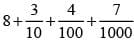Detailed Solution for Olympiad Test : Decimals - Question 6

Given expression can be written as
8 + 0.3 + 0.04 + 0.007 = 8.347

Olympiad Test : Decimals - Question 7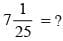Detailed Solution for Olympiad Test : Decimals - Question 7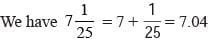Olympiad Test : Decimals - Question 8

6 – 0.23 + 1.2 – 5.76 = ?

Detailed Solution for Olympiad Test : Decimals - Question 8

? = 6 – 0.23 + 1.2 – 5.76
= 7.2 – 5.99 = 1.21

Olympiad Test : Decimals - Question 9

37 – 35.79 = ?

Detailed Solution for Olympiad Test : Decimals - Question 9

? = 37 – 35.79 = 1.21

Olympiad Test : Decimals - Question 10

What is to be added to 74.5 to get 81?

Detailed Solution for Olympiad Test : Decimals - Question 10

Required number = 81 – 74.5 = 6.5

Olympiad Test : Decimals - Question 11

What is to be subtracted from 7.3 to get 0.867?

Detailed Solution for Olympiad Test : Decimals - Question 11

Required number = 7.3 – 0.867 = 6.433

Olympiad Test : Decimals - Question 12

By how much should 79.5 be decreased to get 27.89 ?

Detailed Solution for Olympiad Test : Decimals - Question 12

Required number = 79.5 – 27.89 = 51.61

Olympiad Test : Decimals - Question 13

By how much should 32.754 by increased to get 53?

Detailed Solution for Olympiad Test : Decimals - Question 13

Required number = 53 – 32.754 = 20.246

Olympiad Test : Decimals - Question 14

76.3 – 7.666 – 6.77 – 5.55 = ?

Detailed Solution for Olympiad Test : Decimals - Question 14

? = 76.3 – 7.666 – 6.77 – 5.55
= 76.3 – 19.986 = 56.314

Olympiad Test : Decimals - Question 15

37 mm = ?

Detailed Solution for Olympiad Test : Decimals - Question 15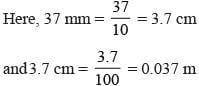Olympiad Test : Decimals - Question 16

2 kg 57 g = ?

Detailed Solution for Olympiad Test : Decimals - Question 16

2 kg 57 g = 2 × 1000 + 57 = 2057 g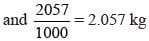Olympiad Test : Decimals - Question 17

During three days of a week, an auto driver earns Rs 302.80, Rs 379.20 and Rs 297.60 respectively. What is his total earning during these days?

Detailed Solution for Olympiad Test : Decimals - Question 17

Total earning = 302.80 + 379.20 + 297.60 = 979.60

Olympiad Test : Decimals - Question 18

What is the decimal equivalent of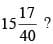Detailed Solution for Olympiad Test : Decimals - Question 18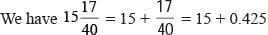= 15.425

Olympiad Test : Decimals - Question 19

Mohan purchased 5 kg 75 g of fruits and 3kg 465g of vegetables and put them in a bag. If this bag with these contents weights 9 kg. What is the weight of empty bag?

Detailed Solution for Olympiad Test : Decimals - Question 19

Here, 5kg 75g + 3kg 465g = 8kg 540g
∴ 9kg - 8kg 540g = 460g

Olympiad Test : Decimals - Question 20

What should be subtracted from the sum of 4.902 & 15.376 to get 16.307 ?

Detailed Solution for Olympiad Test : Decimals - Question 20

Here, 4.902 + 15.376 = 20.278
then 20.278 – 16.307 = 3.971

## Maths Olympiad Class 6

43 tests
 Use Code STAYHOME200 and get INR 200 additional OFF Use Coupon Code
Information about Olympiad Test : Decimals Page
In this test you can find the Exam questions for Olympiad Test : Decimals solved & explained in the simplest way possible. Besides giving Questions and answers for Olympiad Test : Decimals, EduRev gives you an ample number of Online tests for practice

43 tests